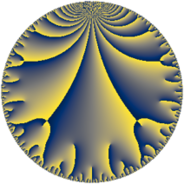Properties

 Label 91.10.rLevel $91$ Weight $10$ Character orbit 91.r Rep. character $\chi_{91}(25,\cdot)$ Character field $\Q(\zeta_{6})$ Dimension $164$ Newform subspaces $1$ Sturm bound $93$ Trace bound $0$

Related objects

Defining parameters

 Level: $$N$$ $$=$$ $$91 = 7 \cdot 13$$ Weight: $$k$$ $$=$$ $$10$$ Character orbit: $$[\chi]$$ $$=$$ 91.r (of order $$6$$ and degree $$2$$) Character conductor: $$\operatorname{cond}(\chi)$$ $$=$$ $$91$$ Character field: $$\Q(\zeta_{6})$$ Newform subspaces: $$1$$ Sturm bound: $$93$$ Trace bound: $$0$$

Dimensions

The following table gives the dimensions of various subspaces of $$M_{10}(91, [\chi])$$.

Total New Old
Modular forms 172 172 0
Cusp forms 164 164 0
Eisenstein series 8 8 0

Trace form

 $$164 q - 326 q^{3} + 20478 q^{4} - 513456 q^{9} + O(q^{10})$$ $$164 q - 326 q^{3} + 20478 q^{4} - 513456 q^{9} + 37950 q^{10} + 328586 q^{12} + 205424 q^{13} - 347348 q^{14} - 5516922 q^{16} + 638792 q^{17} - 2021136 q^{22} - 3143742 q^{23} + 29660776 q^{25} - 4349148 q^{26} + 15971728 q^{27} + 11405516 q^{29} + 3318068 q^{30} - 13283874 q^{35} - 268857760 q^{36} + 41257144 q^{38} - 43335142 q^{39} - 64638572 q^{40} + 4428624 q^{42} + 208624280 q^{43} + 365752796 q^{48} - 8021754 q^{49} - 128665496 q^{51} + 116775564 q^{52} + 274651312 q^{53} + 333747724 q^{55} + 13700502 q^{56} + 205860820 q^{61} - 1213186480 q^{62} - 4080539840 q^{64} + 331150788 q^{65} - 1310285310 q^{66} - 598025264 q^{68} - 1943339932 q^{69} + 920056770 q^{74} + 1640230784 q^{75} + 1201037654 q^{77} + 2439213164 q^{78} - 335791710 q^{79} - 4192022058 q^{81} - 617219896 q^{82} - 3240850496 q^{87} + 1326885718 q^{88} + 14332940596 q^{90} - 3162562236 q^{91} - 7831634604 q^{92} + 1733533760 q^{94} + 319654502 q^{95} + O(q^{100})$$

Decomposition of $$S_{10}^{\mathrm{new}}(91, [\chi])$$ into newform subspaces

Label Dim. $$A$$ Field CM Traces $q$-expansion
$a_{2}$ $a_{3}$ $a_{5}$ $a_{7}$
91.10.r.a $164$ $46.868$ None $$0$$ $$-326$$ $$0$$ $$0$$